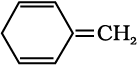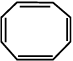Question 13.12:-

Explain why the following systems are not aromatic?

(i)(ii)(iii)(i)For the given compound, the number of π-electrons is 6.
By Huckel’s rule,
4n + 2 = 6
4n = 4  = 1
For a compound to be aromatic, the value of n must be an integer (n = 0, 1, 2…). Since the value of n is an integer, the given compound is aromatic in nature.
(ii)For the given compound, the number of π-electrons is 4.
By Huckel’s rule,
4n + 2 = 4
4n = 2
$n=\frac{1}{2}$
For a compound to be aromatic, the value of n must be an integer (n = 0, 1, 2…), which is not true for the given compound. Hence, it is not aromatic in nature.
(iii)For the given compound, the number of π-electrons is 8.
By Huckel’s rule,
4n + 2 = 8
4n = 6
$n=\frac{3}{2}$
For a compound to be aromatic, the value of n must be an integer (n = 0, 1, 2…). Since the value of n is not an integer, the given compound is not aromatic in nature.